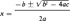Phrases starting with the letter: A B C D E F G H I J K L M N O P Q R S T U V W X Y Z

1. Pertaining to or resembling a square.
2. Relating to a quadratic equation.
noun Math.

1. An equation of the second degree. It is a pure, simple, or incomplete quadratic when it contains only the second power of the variable, as ax2 + c = O; a complete or adfected quadratic when it contains also the first power, as ax2 + bx + c = O.
2. A formula, x, for computing the roots of the standard quadratic equation, ax2 + bx + c = O.
3. plural The part of algebra that treats of quadratic equations.